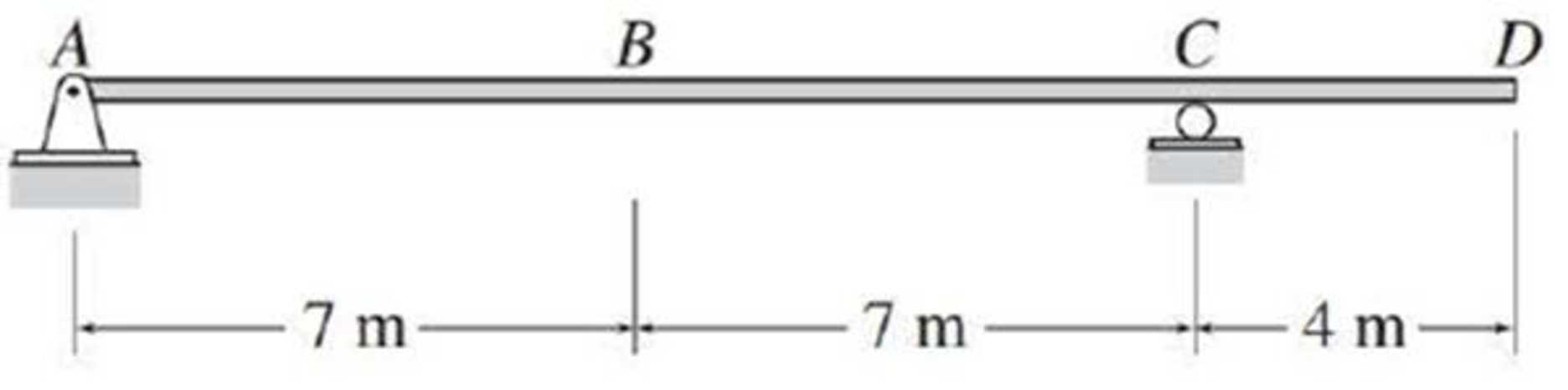# For the beam of Problem 8.6, determine the maximum upward reaction at support A due to a 35 kN/m uniformly distributed live load. FIG. P8.6, P8.61

#### Solutions

Chapter
Section
Chapter 9, Problem 2P
Textbook Problem
13 views

## For the beam of Problem 8.6, determine the maximum upward reaction at support A due to a 35 kN/m uniformly distributed live load.FIG. P8.6, P8.61

To determine

Find the maximum upward reaction at support A.

### Explanation of Solution

Given Information:

The uniformly distributed live load (w) is 35kN/m.

Calculation:

Apply a 1 kN unit moving load at a distance of x from left end A.

Sketch the free body diagram of beam as shown in Figure 1.

Refer Figure 1.

Find the equation of support reaction (Cy) at C using equilibrium equation:

Consider moment equilibrium at point C.

Consider clockwise moment as positive and anticlockwise moment as negative.

Sum of moment at point C is zero.

ΣMC=0Ay(14)1(14x)=014Ay=14xAy=1x14        (1)

Consider Equation (1).

Find the value of influence line ordinate of reaction Ay at support A.

Substitute 0 for x in Equation (2).

Ay=1014=1kN/kN

Similarly calculate the influence line ordinate of reaction Ay for different value of x and summarize the result in Table 1.

 x (m) Ay (kN/kN) 0 1 14 0 18 −27

Draw the influence line diagram for the vertical reactions at support A using Table 1 as shown in Figure 2

### Still sussing out bartleby?

Check out a sample textbook solution.

See a sample solution

#### The Solution to Your Study Problems

Bartleby provides explanations to thousands of textbook problems written by our experts, many with advanced degrees!

Get Started

Find more solutions based on key concepts
Use the data given in Figure 14.10 and duplicate the chart shown there.

Engineering Fundamentals: An Introduction to Engineering (MindTap Course List)

List uses of a personal cloud.

Enhanced Discovering Computers 2017 (Shelly Cashman Series) (MindTap Course List)

What is a cost-benefit analysis?

Principles of Information Security (MindTap Course List)

What is the purpose of postheating the metal after welding?

Welding: Principles and Applications (MindTap Course List)

If your motherboard supports ECC DDR3 memory, can you substitute non-ECC DDR3 memory?

A+ Guide to Hardware (Standalone Book) (MindTap Course List)